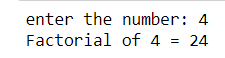# Python 3 Program To Find The Factorial Of A Number

3025

Here you will learn a simple Python 3 program to find the factorial of a number. Before moving ahead, make sure that you have a basic knowledge of Python programming language concepts such as syntax, variables, if-else statements, and for loops. In case you are looking for an excellent book to learn Python, then check out our list of recommended Python books for beginners.

## Python 3 Program To Find The Factorial Of A Number

To calculate the factorial of a number, you first have to take input from the user and then check if the number is positive or negative. If the number is positive, you can use the for loop to calculate the factorial of that number. Check out this self-explanatory Python code.

```fact = 1
num = int(input("enter the number"))
if num < 0:
print("Enter a +ve number")
elif num == 0:
print("0 factorial =1")
else:
for i in range(1, num + 1):
fact = fact * i
print("Factorial of", num, "=", fact)```

### Output

Enter the number: 4
Factorial of 4 = 24